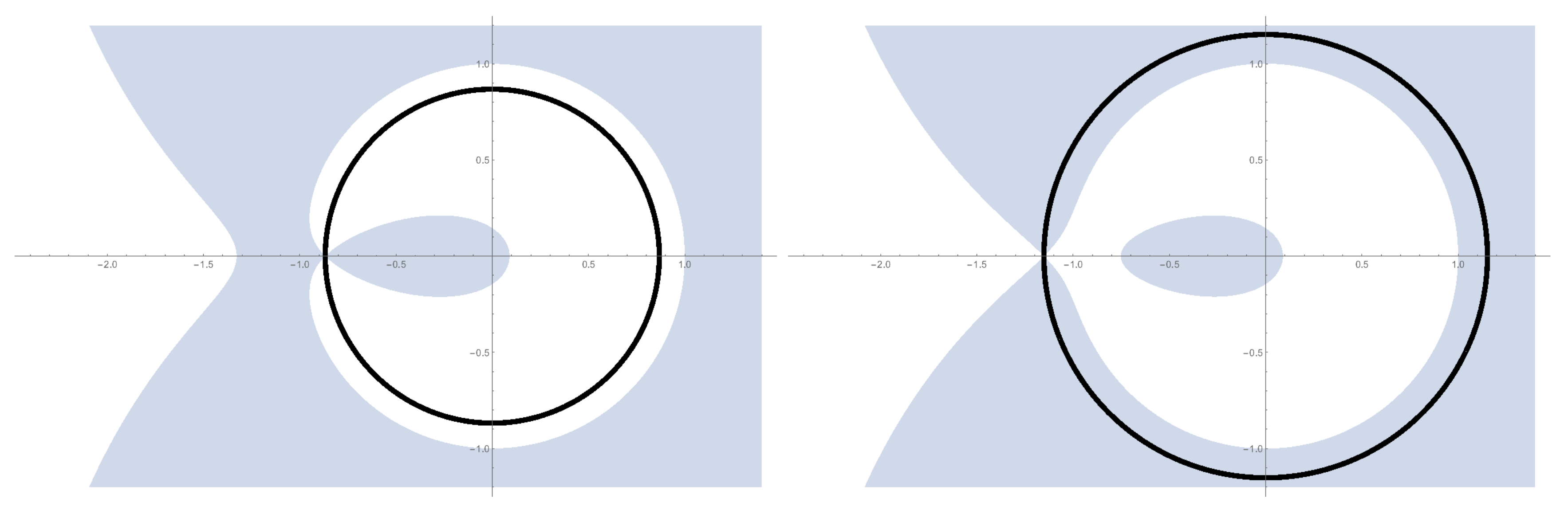# Coarsening model on $\mathbb{Z}^{d}$ with biased zero-energy flips and an exponential large deviation bound for ASEP

### 2017/08/17

(with Michael Damron, David Sivakoff)
Communications in Mathematical Physics 362 (2018), no. 1, 185–217arXiv:1708.05806 [math.PR]

We study the coarsening model (zero-temperature Ising Glauber dynamics) on $\mathbb{Z}^d$ (for $d \geq 2$) with an asymmetric tie-breaking rule. This is a Markov process on the state space ${-1,+1}^{\mathbb{Z}^d}$ of “spin configurations” in which each vertex updates its spin to agree with a majority of its neighbors at the arrival times of a Poisson process. If a vertex has equally many $+1$ and $-1$ neighbors, then it updates its spin value to $+1$ with probability $q \in [0,1]$ and to $-1$ with probability $1-q$. The initial state of this Markov chain is distributed according to a product measure with probability $p$ for a spin to be $+1$.

In this paper, we show that for any given $p>0$, there exist $q$ close enough to 1 such that a.s. every spin has a limit of $+1$. This is of particular interest for small values of $p$, for which it is known that if $q=1/2$, a.s. all spins have a limit of $-1$. For dimension $d=2$, we also obtain near-exponential convergence rates for $q$ sufficiently large, and for general $d$, we obtain stretched exponential rates independent of $d$. Two important ingredients in our proofs are refinements of block arguments of Fontes-Schonmann-Sidoravicius and a novel exponential large deviation bound for the Asymmetric Simple Exclusion Process.This is how the GUE Tracy-Widom/Airy$_2$ double critical point is deformed in our large deviations regime. In this case the double critical point is split into two real critical points, and the large deviations function comes from the difference between the values of $S(\cdot)$ at these two new points